﻿ A Generalized Poisson-Akash Distribution: Properties and ApplicationsInternational Journal of Statistics and Applications

p-ISSN: 2168-5193    e-ISSN: 2168-5215

2018;  8(5): 249-258

doi:10.5923/j.statistics.20180805.03### A Generalized Poisson-Akash Distribution: Properties and Applications

Rama Shanker1, Kamlesh Kumar Shukla1, Tekie Asehun Leonida2

1Department of Statistics, College of Science, Eritrea Institute of Technology, Asmara, Eritrea

2Department of Applied Mathematics, University of Twente, The Netherlands

Correspondence to: Rama Shanker, Department of Statistics, College of Science, Eritrea Institute of Technology, Asmara, Eritrea.
 Email: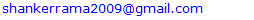Abstract

A generalized Poisson- Akash distribution which includes Poisson-Akash distribution has been proposed. Its factorial moments, raw moments and central moments have been derived and studied. Some statistical properties including generating functions, hazard rate function and unimodality have been discussed. Method of moments and the method of maximum likelihood have been discussed for estimating parameters of the distribution. Applications of the proposed distribution have been explained through two count datasets and compared with other discrete distributions.

Keywords: Generalized Akash distribution, Poisson- Akash distribution, Compounding, Moments, Skewness, Kurtosis, Maximum likelihood estimation, Applications

Cite this paper: Rama Shanker, Kamlesh Kumar Shukla, Tekie Asehun Leonida, A Generalized Poisson-Akash Distribution: Properties and Applications, International Journal of Statistics and Applications, Vol. 8 No. 5, 2018, pp. 249-258. doi: 10.5923/j.statistics.20180805.03.

### 1. Introduction

The statistical analysis and modeling of count data are crucial in almost all fields of knowledge including biological science, insurance, medical science, finance, sociology, psychology, are some among others. Count data are generated by many phenomena such as the number of insurance claimants in insurance, number of yeast cells in biological science, number of chromosomes in genetics, etc. It has been observed that, in general, count data follows under-dispersion (variance < mean), equi-dispersion (variance = mean) or over-dispersion (variance > mean). The over-dispersion of count data have been addressed using mixed Poisson distributions by different researchers including Raghavachari et al (1997), Karlis and Xekalaki (2005), Panjeer (2006), some among others. Mixed Poisson distributions arise when the parameter of the Poisson distribution is a random variable having some specified distributions. The distribution of the parameter of the Poisson distribution is known as mixing distribution. It has been observed that the general characteristics of the mixed Poisson distribution follow some characteristics of its mixing distributions. Various mixed Poisson distributions have been derived in statistics by selecting different mixing distribution.
The classical negative binomial distribution (NBD) derived by Greenwood and Yule (1920) is the mixed Poisson distribution where the parameter of the Poisson random variable is distributed as a gamma random variable. The NBD has been used to model over-dispersed count data. However, the NBD may not be suitable for some over-dispersed count data due to its theoretical or applied point of view. Other mixed Poisson distributions arise from alternative mixing distributions. For example, the Poisson-Lindley distribution, introduced by Sankaran (1970), is a Poisson mixture of Lindley (1958) distribution. The Poisson-Akash distribution, introduced by Shanker (2017), is a Poisson mixture of Akash distribution proposed by Shanker (2015. It has been observed by Karlis and Xekalaki (2005) that there are naturally arising situations where a good fit is not obtainable with a particular mixed Poisson distribution in case of over-dispersed count data. This shows that there is a need for new mixed Poisson distribution which gives a better fit as compared with the existing mixed Poisson distributions.
Shanker (2017) introduced the discrete Poisson- Akash distribution (PAD) to model count data and defined by its probability mass function (pmf)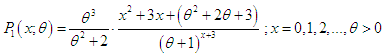(1.1)
Moments and moments based measures, statistical properties; estimation of parameter using both the method of moments and the method of maximum likelihood and applications of PAD has been discussed by Shanker (2017). The distribution arises from the Poisson distribution when its parameter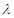follows Akash distribution introduced by Shanker (2015) and defined by its probability density function (pdf)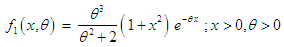(1.2)
The pdf (1.2) is a convex combination of exponential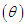and gamma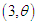distributions. Shanker (2015) discussed statistical properties including moments based coefficients, hazard rate function, mean residual life function, mean deviations, stochastic ordering, Renyi entropy measure, order statistics, Bonferroni and Lorenz curves, stress- strength reliability, along with estimation of parameter and applications to model lifetime data from biomedical science and engineering.
The first four moments about origin and the variance of PAD (1.1) obtained by Shanker (2017) are given by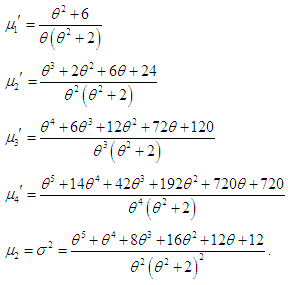Recently Shanker et al (2018) proposed a generalized Akash distribution (GAD) having parameters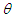and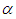and defined by its pdf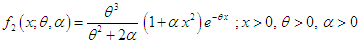(1.3)
Its structural properties including moments, hazard rate function, mean residual life function, mean deviations, stochastic ordering, Renyi entropy measure, order statistics, Bonferroni and Lorenz curves, stress- strength reliability, estimation of parameters and applications for modeling survival time data has been discussed by Shanker et al (2018). It can be easily shown that at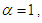GAD (1.3) reduces to Akash distribution (1.2).
The main purpose of this paper is to introduce a generalized Poisson- Akash distribution, a Poisson mixture of generalized Akash distribution proposed by Shanker et al (2018). Its moments based measures including coefficients of variation, skewness, kurtosis and index of dispersion have been derived and their nature and behavior has been discussed graphically. Its statistical properties including generating functions, hazard rate function and unimodality have been discussed. The estimation of parameters has been discussed using method of moments and the method of maximum likelihood. Applications and goodness of fit of the distribution have also been discussed through two examples of observed real count datasets and the fit has been found quite satisfactory over other discrete distributions.

### 2. A Generalized Poisson- Akash Distribution

Assuming that the parameter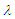of the Poisson distribution follows GAD (1.3), the Poisson mixture of GAD can be obtained as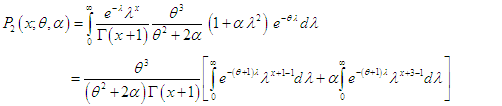(2.1)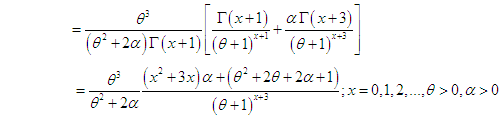(2.2)
We would call this pmf a generalized Poisson - Akash distribution (GPAD). It can be easily verified that PAD (1.1) is a particular case of GPAD for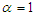. The nature and behavior of GPAD for varying values of the parametersandhave been explained graphically in figure 1.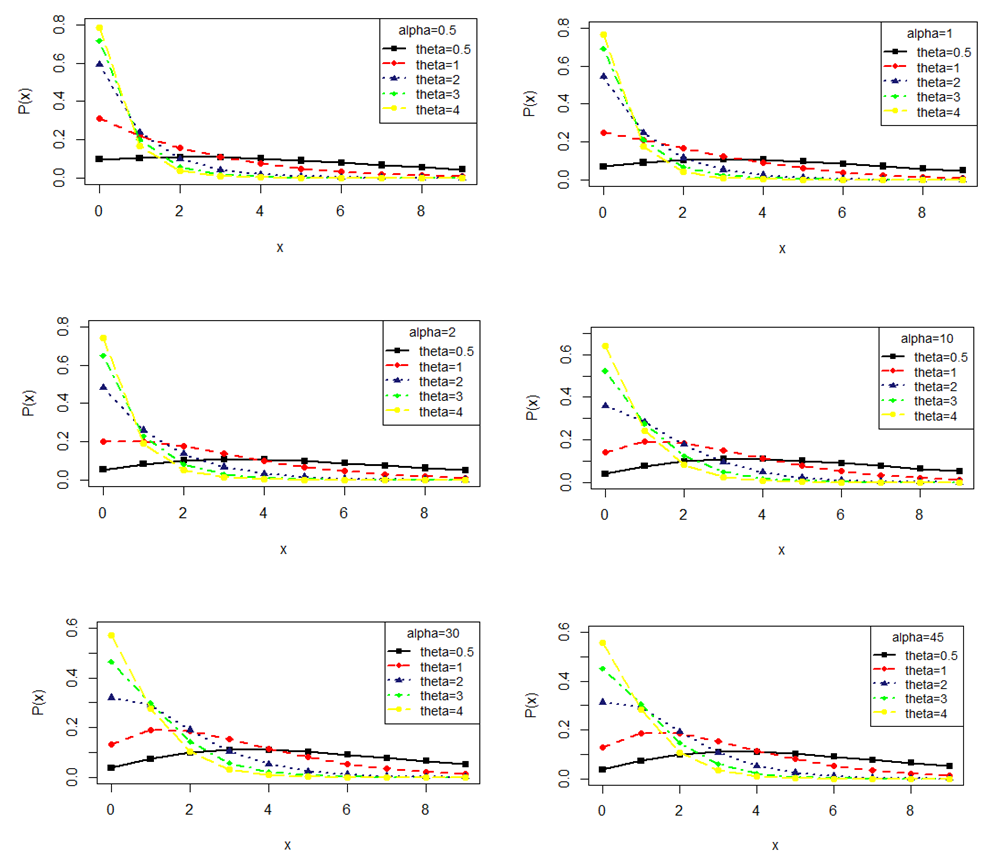Figure 1. Probability mass function plot of GPAD for varying values of parameters θ and α

### 3. Moments

#### 3.1. Factorial Moments

Using (2.1), the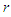th factorial moment about origin of the GPAD (2.2) can be obtained as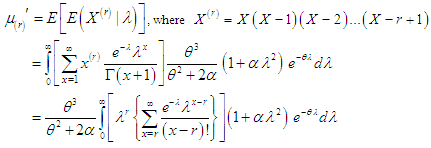Taking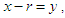we get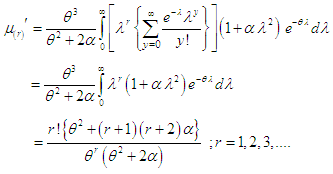(3.1.1)
Taking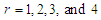in (3.1.1), the first four factorial moments about origin of GPAD (2.2) can be obtained as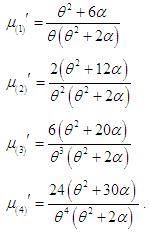#### 3.2. Moments about Origin (Raw moments)

The first four moments about origin, using the relationship between factorial moments about origin and the moments about origin, of GPAD (2.2) can be obtained as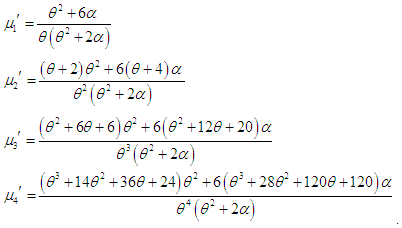#### 3.3. Moments about the Mean (Central moments)

Using the relationship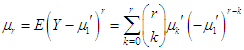between moments about the mean and the moments about origin, the moments about the mean of the GPAD (2.2) can be obtained as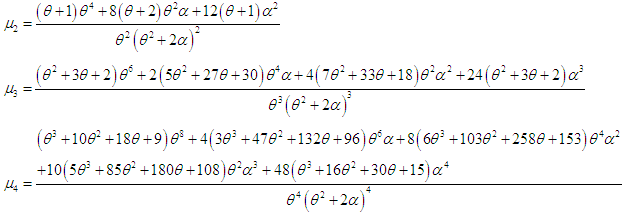### 4. Coefficient of Variation, Skewness, Kurtosis and Index of Dispersion

The coefficient of variation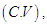coefficient of Skewness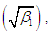coefficient of Kurtosis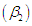and index of dispersion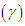of the GPAD (2.2) are thus obtained as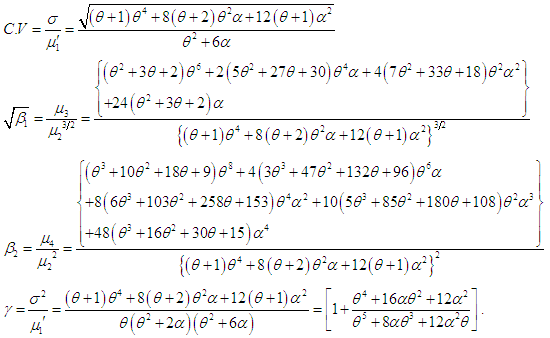Now from the index of dispersion it is obvious that if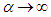and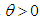, then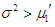(over dispersion) and hence GPAD is a suitable model for over dispersed data. Nature and behavior of coefficient of variation, coefficient of skewness, coefficient of kurtosis and index of dispersion of GPAD for varying values of parameters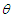and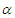have been shown graphically in figure 2.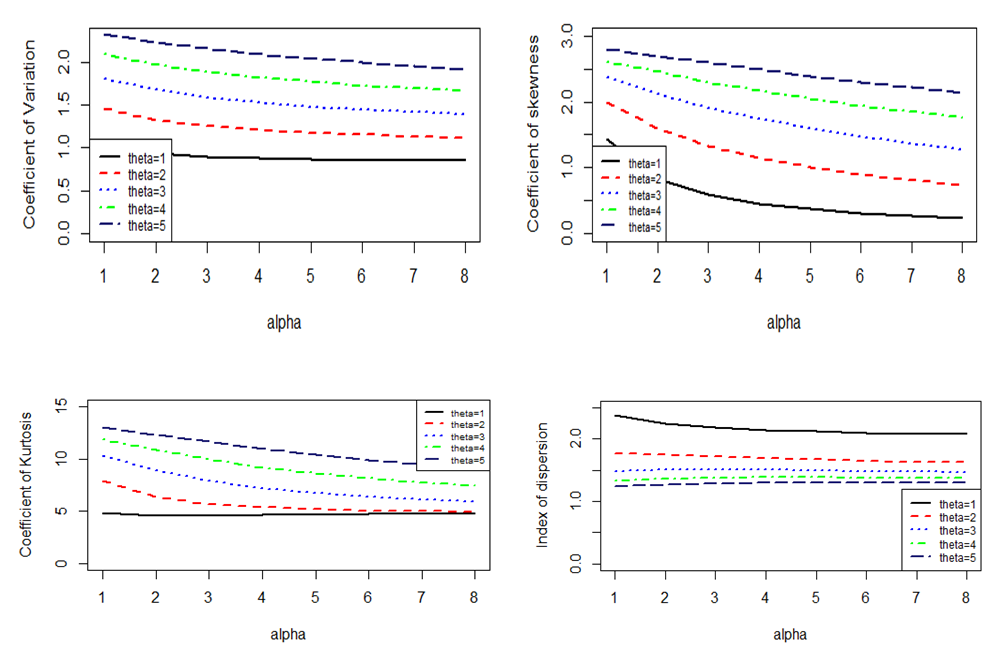Figure 2. Nature and behavior of coefficient of variation, coefficient of skewness, coefficient of kurtosis and index of dispersion of GPAD for varying values of parameters θ and α

### 5. Statistical Properties

#### 5.1. Generating Functions

The probability generating function of GPAD can be obtained as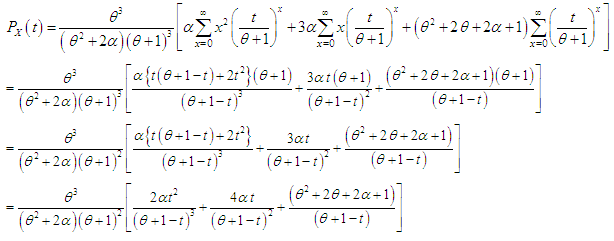The moment generating function of GPAD is thus given by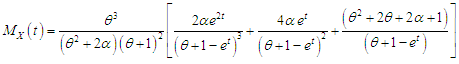#### 5.2. Increasing Hazard Rate and Unimodality

We have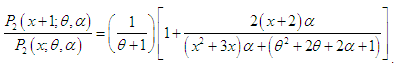It can be easily verified that this is a decreasing function in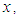and hence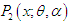is log-concave. Now using the results of relationship between log-concavity, unimodality and increasing hazard rate (IHR) of discrete distributions available in Grandell (1997), it can concluded that GPAD (2.2) has an increasing hazard rate and is unimodal.

### 6. Parameter Estimation

#### 6.1. Method of Moments

Since GPAD has two parameters to be estimated, taking the first two moments about origin, we get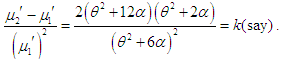Assuming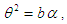we get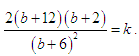This gives a quadratic equation in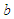as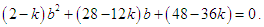Replacing the first population moment about origin and the second population moment about origin with their respective sample moments, an estimate of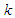can be obtained and substituting the value of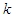in the above equation, an estimate of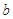can be obtained. Again, replacing the population mean with the corresponding sample mean and taking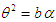in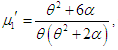we get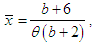which gives MOME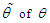as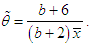Thus MOME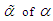can be expressed as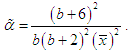#### 6.2. Maximum Likelihood Estimation

Let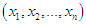be a random sample of size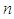from the GPAD (2.2) and let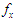be the observed frequency in the sample corresponding to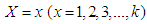such that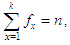where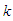is the largest observed value having non-zero frequency. The log- likelihood function of GPAD (2.2) can be given by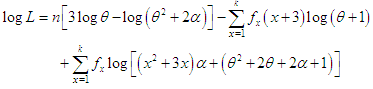The maximum likelihood estimates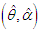of parameters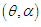of GPAD (2.2) is the solutions of the following log- likelihood equations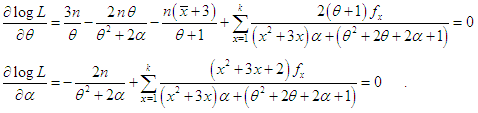where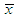is the sample mean. These two log- likelihood equations do not seem to be solved directly because they are not in closed forms. These two log-likelihood equations can be solved iteratively using R-software till sufficiently close values of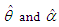are obtained. The initial values of parameters are taken as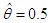and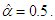### 7. Applications

The GPAD has been fitted to two count datasets from biological sciences to test its goodness of fit over Poisson distribution (PD), Poisson-Lindley distribution (PLD) and Poisson-Akash distribution (PAD). The maximum likelihood estimate (MLE) has been used to fit the GPAD. The first dataset is the number of Student’s historic data on Haemocytometer counts of yeast cells available in Gosset (1908) and the second data set is the number of European corn- borer of Mc. Guire et al (1957). The fitted plots of distributions for datasets in tables 1 and 2 are presented in figure 3. It is clear from the goodness of fit of GPAD and from the fitted plots of distributions that GPAD gives much closer fit than PD, PLD, and PAD and hence it can be considered as an important distribution in ecology.
 Table 1. Observed and expected number of Haemocytometer yeast cell counts per square observed by Gosset (1908)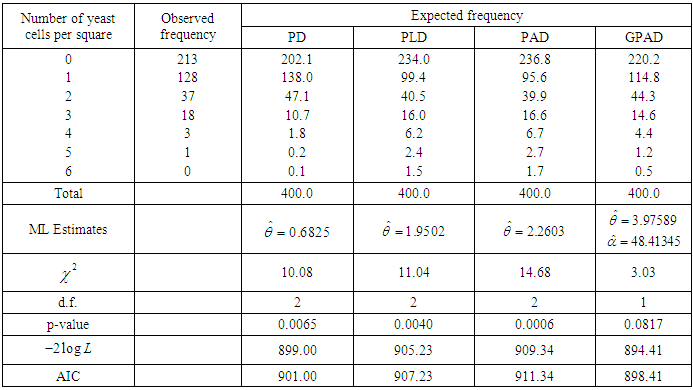Table 2. Observed and expected number of European corn- borer of Mc. Guire et al (1957)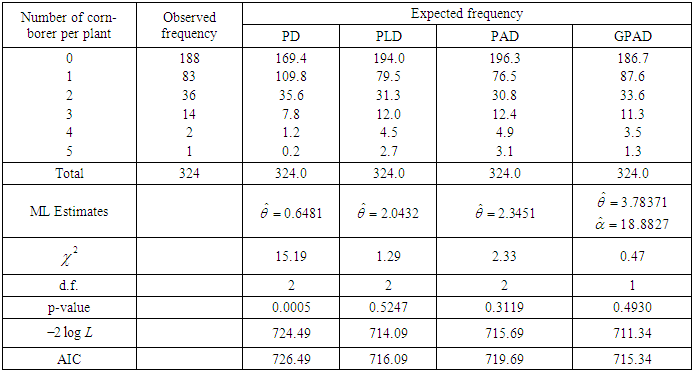The fitted plot of distributions for datasets in table 1 and 2 are shown in figure 3. It is obvious from the goodness of fit in tables 1 and 2 and the fitted plot of distributions in figure 3 that GPAD gives better fit.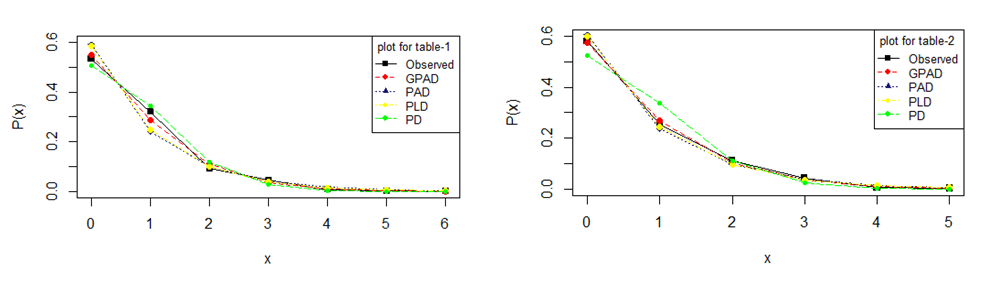Figure 3. Fitted plots of distributions for datasets 1 and 2

### 8. Concluding Remarks

This paper proposes a generalized Poisson- Akash distribution which includes Poisson-Akash distribution as a particular case. Its moments and moments based measures have been derived and studied. Some statistical properties have been discussed. Method of moments and the method of maximum likelihood have been discussed for estimating parameters of the distribution. Finally, applications of the proposed distribution have been explained through two count datasets from biological sciences and the goodness of fit has been found quite satisfactory over PD, PLD and PAD.

### ACKNOWLEDGEMENTS

Authors are grateful to the editor in chief and the anonymous reviewer for their constructive comments which enhanced the quality of the paper.

### References

  Grandell, J. (1997): Mixed Poisson Processes, Chapman & Hall, London.  Greenwood, M. and Yule, G.U. (1920): An inquiry into the nature of frequency distributions representative of multiple happenings with particular reference to the multiple attacks of disease or of repeated accidents, Journal of the Royal Statistical Society , 83(2), 115-121.  Gosset, W.S. (1908): The probable error of a mean, Biometrika, 6, 1-25.  Karlis, D. and Xekalaki, E. (2005): Mixed Poisson distributions, International Statistical review, 73(1), 35-58.  Lindley, D.V. (1958): Fiducial distributions and Bayes theorem, Journal of the Royal Statistical Society, 20 (1), 102-107.  Mc. Guire, J.U., Brindley, T.A. and Bancroft, T.A. (1957): The distribution of European corn-borer larvae pyrausta in field corn, Biometrics, 13, 65-78.  Panjeer, H. H. (2006): Mixed Poisson distributions. In Encyclopedia of Actuarial Science, John Wiley and Sons Ltd, Hoboken, New Jersey, USA.  Raghavachari, M., Srinivasam, A. and Sullo, P. (1997): Poisson mixture yield models for integrated circuits – A critical review, Microelectronics Reliability, 37 (4), 565-580.  Sankaran, M. (1970): The discrete Poisson-Lindley distribution, Biometrics, 26, 145-149.  Shanker, R. (2015): Akash distribution and Its Applications, International Journal of Probability and Statistics, 4(3), 65-75.  Shanker, R. (2017): The Discrete Poisson-Akash Distribution, International Journal of Probability and Statistics, 6(1), 1-10.  Shanker, R. Shukla, K.K., Shanker, R., Singh, A.P (2018): A Generalized Akash Distribution, Biometrics & Biostatistics International Journal, 7(1), 1-10.